# Odds and Probabilities

Throughout this website, I am going to use terms like "odds" and "probabilities." These terms can be expressed as ratios, percentages or fractions and each has a slightly different meaning. Just in case you slept through your course Statistics 101, or even managed to escape the experience altogether, I am going to attempt to clear things up.

### Our Favorites Casino sites for 2023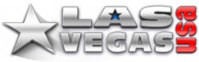### Bonus up to \$3,000

The best daily bonuses on the internet

Our score: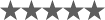(4.3 / 5)### 150% up to \$10,000

Welcome Bonus

Our score:(4.2 / 5)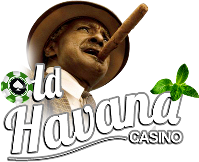### 250% Bonus up to \$1,000

Welcome Bonus

Our score:(4.2 / 5)### 400% up to \$10,000

Bonus Code: 400BONUS

Our score:(4.2 / 5)### 400% up to \$10,000

Bonus Code: 400BONUS

Our score:(4.2 / 5)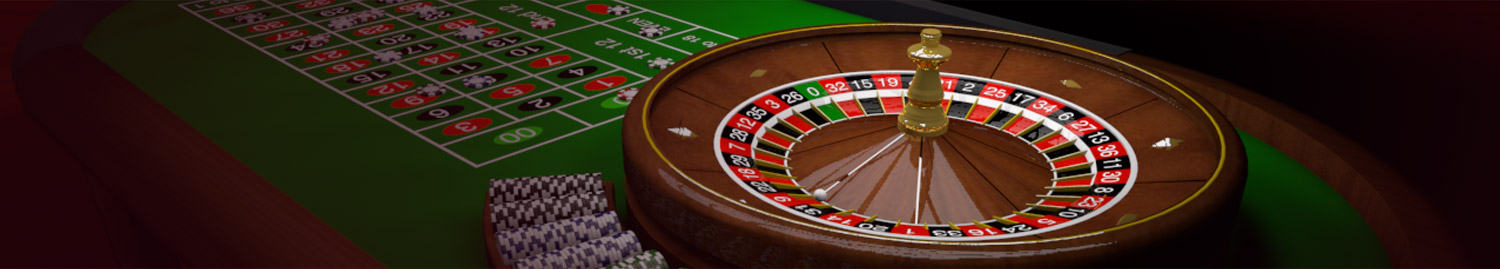## Gambling & Probability

A general definition of probability is the likelihood that a given event will occur. When we apply this concept to gambling, we usually end up with a specific expression like 1 out of 2 or 1:2.

When the probability is expressed as two numbers, the first number represents the expected frequency of a specific event occurring. The second number is the total number of possible events or outcomes, including the specific event and all other events that can occur.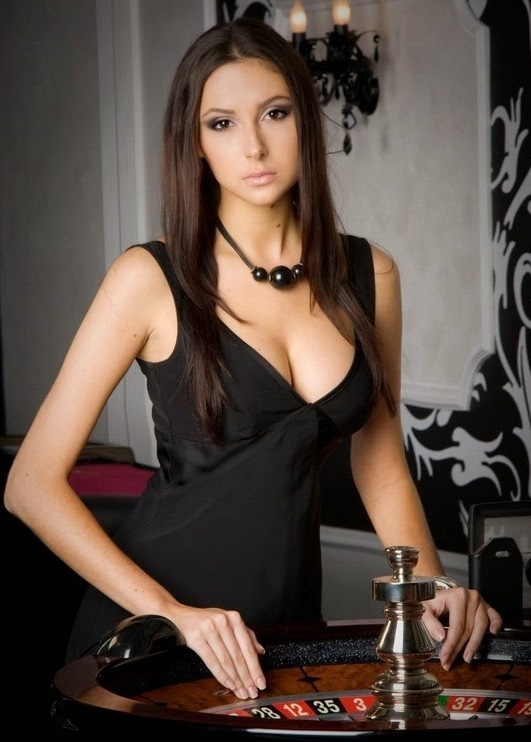Let's take a look at the concept of probability applied to coin flips. Consider this question - "What is the probability of a head showing on the next coin flip?

Since there are two possible outcomes (heads or tails) and we are looking for only one event (heads), this probability can be expressed as 1 out of 2, 1 to 2 or 1:2 or even 1/2.

This two number expression can also be converted to a percentage by dividing the first number by the second number, which, in this case, will give us: 1 divided by 2 equals 0.50, which can also be expressed as 50%.

Therefore, the probability of getting heads on the next coin flip is 1 to 2 or 50%.

Let's apply this concept to the game of roulette. The American version of the game has the numbers 1 to 36, plus a zero and a double-zero, for a total of 38 numbers. This gives us 38 possible outcomes on any spin of the wheel. If your favorite number is 17, and you wanted to know how likely this was to show on the next spin of the wheel, you could express this as 1 out of 38, 1 to 38, 1:38 or 1/38.

To determine this probability as a percentage means - 1 divided by 38 equals 0.026 which is 2.6% as a percentage.

Interpretation? There is a 2.6% probability or chance of your wager on the number 17 winning on the next spin of the roulette wheel.